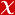## Nuclear modification of Y states in pPb collisions at sNN=5.02TeV

Physics Letters, Section B: Nuclear, Elementary Particle and High-Energy Physics, vol.835, 2022 (SCI-Expanded)#### Abstract

© 2022 The Author(s)Production cross sections of [Formula presented], [Formula presented], and [Formula presented] states decaying into [Formula presented] in proton-lead (pPb) collisions are reported using data collected by the CMS experiment at sNN=5.02TeV. A comparison is made with corresponding cross sections obtained with pp data measured at the same collision energy and scaled by the Pb nucleus mass number. The nuclear modification factor for [Formula presented] is found to be [Formula presented]. Similar results for the excited states indicate a sequential suppression pattern, such that [Formula presented]. The suppression of all states is much less pronounced in pPb than in PbPb collisions, and independent of transverse momentum [Formula presented] and center-of-mass rapidity [Formula presented] of the individual [Formula presented] state in the studied range [Formula presented] and [Formula presented]. Models that incorporate final-state effects of bottomonia in pPb collisions are in better agreement with the data than those which only assume initial-state modifications.